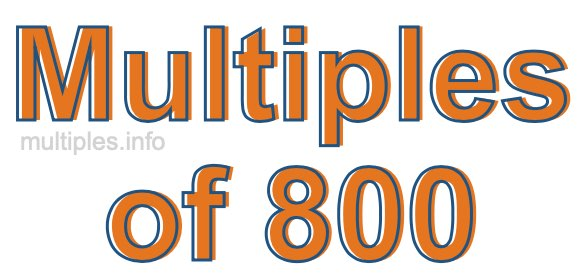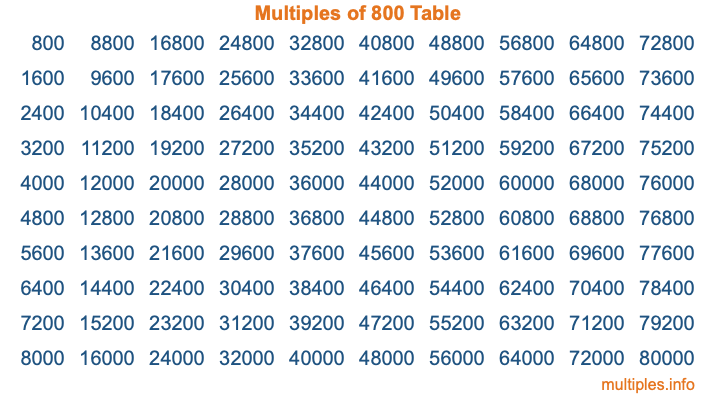Multiples of 800Welcome to the Multiples of 800 page. Here we will first teach you everything you will ever need to know about the multiples of 800, and then give you a study guide summary of everything we taught you to make sure you remember it all. Use this page to look up facts and learn information about the multiples of 800. This page will make you a multiples of eight hundred expert!

Definition of Multiples of 800
Multiples of 800 are all the numbers that when divided by 800 equal an integer. Each of the multiples of 800 are called a multiple. A multiple of 800 is created by multiplying 800 by an integer.

Therefore, to create a list of multiples of 800, you start with 1 multiplied by 800, then 2 multiplied by 800, then 3 multiplied by 800, and so on for as long as you want. Thus, the list of the first five multiples of 800 is 800, 1600, 2400, 3200, and 4000. To see a larger list of multiples of 800, see the printable image of Multiples of 800 further down on this page. We also have a category where you can choose any nth multiple of 800.

Multiples of 800 Checker
The Multiples of 800 Checker below checks to see if any number of your choice is a multiple of 800. In other words, it checks to see if there is any number (integer) that when multiplied by 800 will equal your number. To do that, we divide your number by 800. If the the quotient is an integer, then your number is a multiple of 800.

Is  a multiple of 800?

Least Common Multiple of 800 and ...
A Least Common Multiple (LCM) is the lowest multiple that two or more numbers have in common. This is also called the smallest common multiple or lowest common multiple and is useful to know when you are adding our subtracting fractions. Enter one or more numbers below (800 is already entered) to find the LCM.

Check out our LCM Calculator if you need more details about the Least Common Multiple or if you need the LCM for different numbers for adding and subtraction fractions.

nth Multiple of 800
As we stated above, 800 is the first multiple of 800, 1600 is the second multiple of 800, 2400 is the third multiple of 800, and so on. Enter a number below to find the nth multiple of 800.

th multiple of 800

Multiples of 800 vs Factors of 800
800 is a multiple of 800 and a factor of 800, but that is where the similarities end. All postive multiples of 800 are 800 or greater than 800. All positive factors of 800 are 800 or less than 800.

Below is the beginning list of multiples of 800 and the factors of 800 so you can compare:

Multiples of 800: 800, 1600, 2400, 3200, 4000, etc.

Factors of 800: 1, 2, 4, 5, 8, 10, 16, 20, 25, 32, 40, 50, 80, 100, 160, 200, 400, 800

As you can see, the multiples of 800 are all the numbers that you can divide by 800 to get a whole number. The factors of 800, on the other hand, are all the whole numbers that you can multiply by another whole number to get 800.

It's also interesting to note that if a number (x) is a factor of 800, then 800 will also be a multiple of that number (x).

Multiples of 800 vs Divisors of 800
The divisors of 800 are all the integers that 800 can be divided by evenly. Below is a list of the divisors of 800.

Divisors of 800: 1, 2, 4, 5, 8, 10, 16, 20, 25, 32, 40, 50, 80, 100, 160, 200, 400, 800

The interesting thing to note here is that if you take any multiple of 800 and divide it by a divisor of 800, you will see that the quotient is an integer.

Multiples of 800 Table
Below is an image of the first 100 multiples of 800 in a table. The table is in chronological order, column by column. The first column has the first ten multiples of 800, the second column has the next ten multiples of 800, and so on.The Multiples of 800 Table is also referred to as the 800 Times Table or Times Table of 800. You are welcome to print out our table for your studies.

Negative Multiples of 800
Although not often discussed or needed in math, it is worth mentioning that you can make a list of negative multiples of 800 by multiplying 800 by -1, then by -2, then by -3, and so on, to get the following list of negative multiples of 800:

-800, -1600, -2400, -3200, -4000, etc.

Multiples of 800 Summary
Below is a summary of important Multiples of 800 facts that we have discussed on this page. To retain the knowledge on this page, we recommend that you read through the summary and explain to yourself or a study partner why they hold true.

There are an infinite number of multiples of 800.

A multiple of 800 divided by 800 will equal a whole number.

800 divided by a factor of 800 equals a divisor of 800.

The nth multiple of 800 is n times 800.

The largest factor of 800 is equal to the first positive multiple of 800.

800 is a multiple of every factor of 800.

800 is a multiple of 800.

A multiple of 800 divided by a divisor of 800 equals an integer.

800 divided by a divisor of 800 equals a factor of 800.

Any integer times 800 will equal a multiple of 800.

Multiples of a Number
Here you can get the multiples of another number, all with the same attention to detail as we did for multiples of 800 on this page.

Multiples of
Multiples of 801
Did you find our page about multiples of eight hundred educational? Do you want more knowledge? Check out the multiples of the next number on our list!